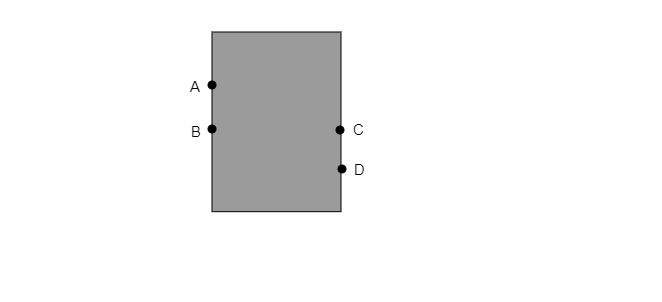" ">

# A water tank has four taps fixed at points A, B, C and D as shown in figure. The water will flow out at the same pressure from taps at$(a)$. B and C $(b)$. A and B $(c)$. C and D $(d)$. A and C"

Since B and C taps are on the same level, so water flows from tap B and tap C at the same pressure. As pressure depends on gravity, density, and height. Here, gravity and density are the same. So, Pressure will depend on the height. Here taps B and C are on the same height.

So, option $(a)$ is correct.

Updated on: 10-Oct-2022

33 Views